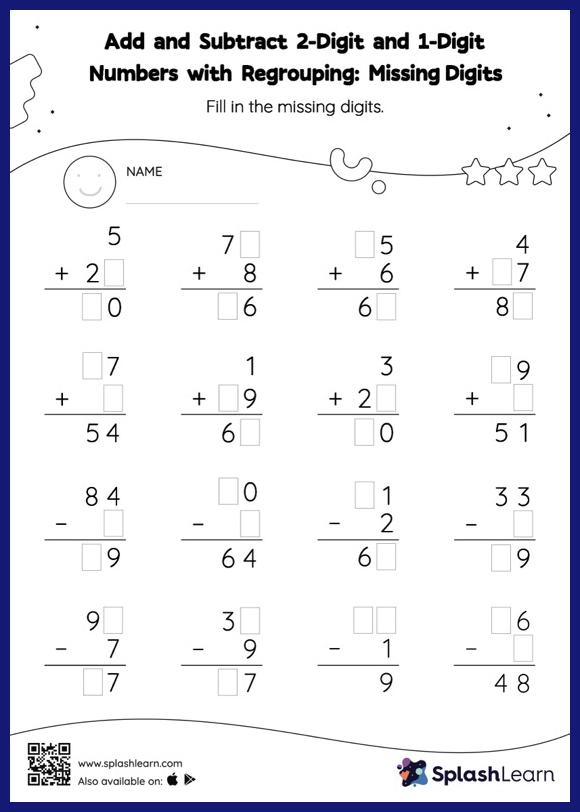# Add and Subtract 2-Digit and 1-Digit Numbers with Regrouping: Missing Digits Worksheet

Home > Add and Subtract 2-Digit and 1-Digit Numbers with Regrouping: Missing DigitsStudents use the relationship between addition and subtraction to find the missing number in this worksheet. They also need to regroup numbers to get to the answer in add and subtract 2-digit and 1-digit numbers with regrouping worksheet. As the worksheet uses the column method, it is helpful in getting students toward higher accuracy, especially with bigger numbers and in scenarios where regrouping is required.